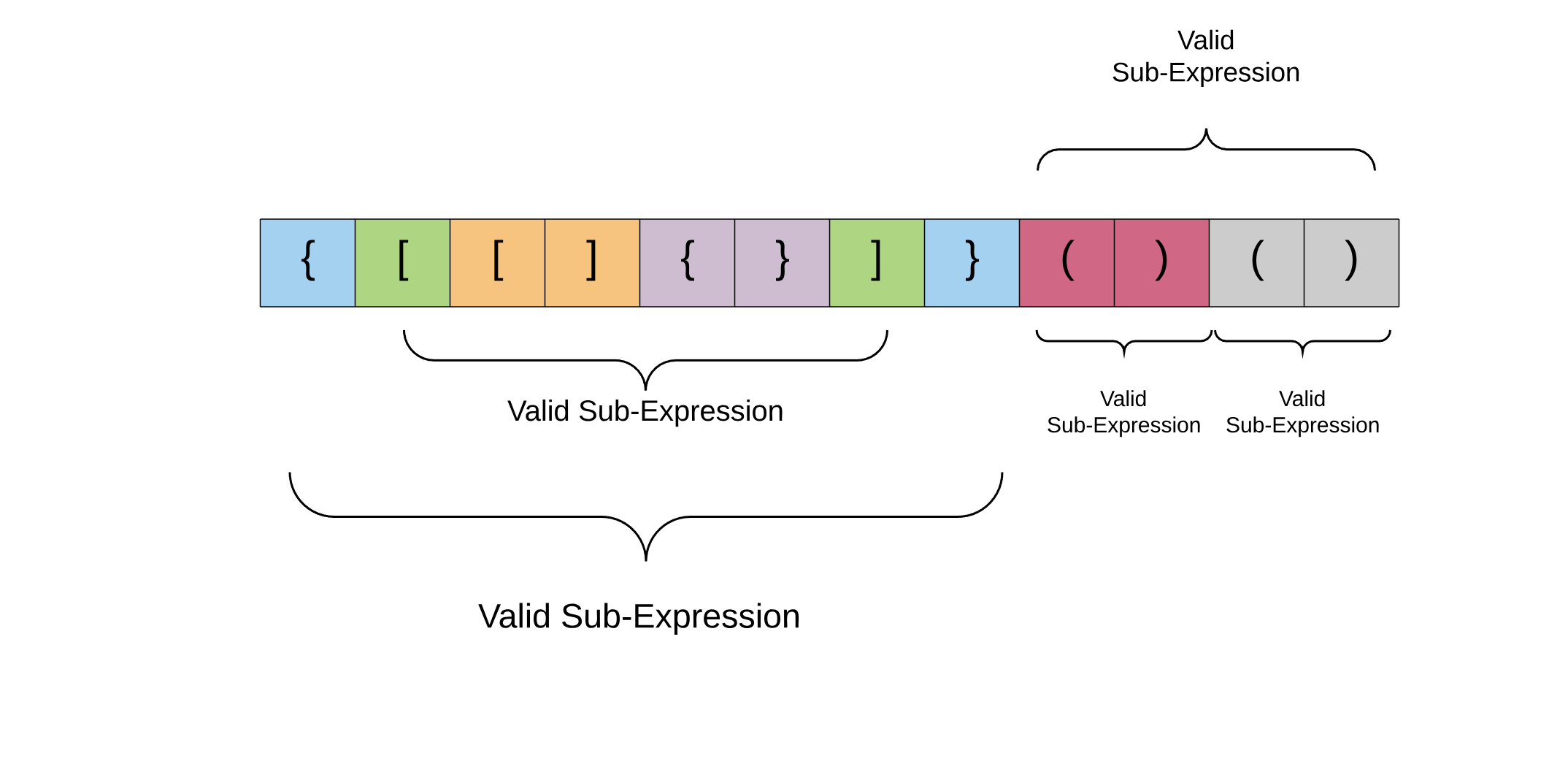## Determine if the input string has valid parentheses combination

The input string is considered as a balanced expression if

1. Open brackets must be closed by the same type of brackets.
2. Open brackets must be closed in the correct order.

Note that an empty String is also considered valid.

```Example 1:
Input: "{()}" → Output: true

Example 2:
Input: "()[]{}" → Output: true

Example 3:
Input: "({]" → Output: false

Example 4:
Input: "([)]" → Output: false

Example 5:
Input: "{[]}" → Output: true```

This solution is very useful for compilers to check if the parentheses are balanced or not. The expressions that we will deal with in this problem can consist of three different types of parenthesis:

• `()`,
• `{}` and
• `[]`

A `Stack` can be used to check whether the given expression has balanced parenthesis. The program will read one character at a time. If the character is an opening delimiter such as (, {, [ - then it is pushed into the `Stack`. When a closing delimiter is encountered like ), } or ] - then the stack is popped and compared against the opening delimiter. If they match then the parsing of the String continues. If they do not match, the program indicates that there is a mismatch of the delimiter. A linear-time algorithm based on the stack can be given as follows.

### Algorithm:

• Create a `Stack`.
• Convert the input String into a `Character` array.
• While the end of the String is not reached, read one character at a time.
• If the character is an opening symbol (, {, [ - then push it into the `Stack`.
• If the character is a closing symbol ), }, ] - then if the stack is empty then return `false`. Otherwise, pop the `Stack.`
• If the popped delimiter does not match with the corresponding character from the String then return `false`. If the current character in the loop is '(' then the popped element must be ')'.
• At the end of the loop if the `Stack` is still not empty then return `false`, which means there are unbalanced parentheses present in the String.

An interesting property about a valid parenthesis expression is that a sub-expression of a valid expression should also be a valid expression.Following is the Java implementation of the algorithm:

``````import java.util.Stack;

public class ParenthesesCheck {

public static void main(String[] args) {
String s = "[])";
System.out.println(isValid(s));
}

public static boolean isValid(String s) {

if (s == null || s.length() == 0) {
return true;
}

char[] arr = s.toCharArray();
Stack<Character> stack = new Stack <>();
for (char inChar: arr) {
if (inChar == '(' || inChar == '{' || inChar == '[') {
stack.push(inChar);
} else {
//')' or '}' or ']' occurred before a opening
if (stack.isEmpty()) {
return false;
}
char fromStack = stack.pop();
if (inChar == ')' && fromStack != '(') {
return false;
} else if (inChar == '}' && fromStack != '{') {
return false;
} else if (inChar == ']' && fromStack != '[') {
return false;
}
}
}
if (!stack.isEmpty()) {
return false;
}
return true;
}
}``````

Complexity analysis

• Time complexity: O(n) because we simply traverse the given string one character at a time and push and pop operations on a stack take O(1) time.
• Space complexity: O(n) as we push all opening brackets onto the stack and in the worst case, we will end up pushing all the brackets onto the stack. e.g. `{[{[(({{`.
Amazon Algorithm Microsoft